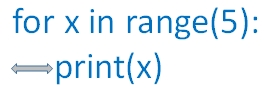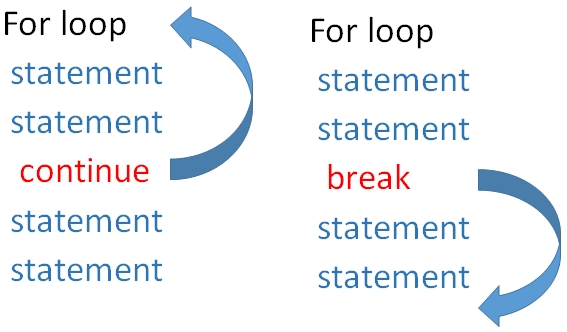# For Loop in Python

For loop with range
``````for x in range(5):
print(x)``````

For loop in python to execute code block repeatedly using continue break and else with nested loopsNote that the 2nd line print(x) is placed after an indente of one space. Without that we will get an error asking expectd an indented block . The code to be executed within the block is to be kept with indenting as there is no end of for loop like other languages.
The output of above code is here
``````0
1
2
3
4``````
Here is a statement which is outside the loop because there is no indenting at the beginning of the line
``````for x in range(5):
print(x)
print("I am outside the loop")
``````
Output is here .
``````0
1
2
3
4
I am outside the loop``````
In the above code the range is from 0 to 5 ( excluding 5 ) , so we get output from 0 to 4.

## Loop with range

Now we will define a range for the loop
``````for x in range(5,10):
print(x)``````
Output is here
``````5
6
7
8
9``````
We can add a increment value
``````for x in range(5,25,5):
print(x)``````
Output is here
``````5
10
15
20``````

## Break and continue in a for loop

When break statement is encountered the execution comes out of the loop. In case of continue the execution returns to the starting of the loop skipping the rest of the statements ( after continue ) and continues again.

In the codes give below , as soon as break is encountered the execution comes out of the loop, so 15 and 20 are not printed. But when continue is used in place of break the printing of 15 is skipped but the loop continues again so 20 is printed.Using continue
``````for x in range(5,25,5):
if(x==15):
continue
print(x)``````
``````5
10
20``````
Using break
``````for x in range(5,25,5):
if(x==15):
break
print(x)``````
``````5
10``````

## Using else with for loop

Here else part of the code is executed once the for loop execution is over. However if loop is terminated by using break then else part of the code is skipped.

Using continue
``````for x in range(5,25,5):
if(x==15):
continue
print(x)
else:
print("Out of loop but with else")
print("Out of loop")``````
Output is here
``````5
10
20
Out of loop but with else
Out of loop``````
Code block inside else will be executed in case of continue but not executed in case of break.

using break
``````for x in range(5,25,5):
if(x==15):
break
print(x)
else:
print("Out of loop but with else")
print("Out of loop")``````
Output is here ( else part is skipped )
``````5
10
Out of loop``````
Check how else in for loop is used to display all prime numbers upto 100.
``````num=100 # change this value to get all prime numbers
for i in range(2,num-1):
for j in range(2,i-1):
if (i%j == 0):
break
else:
print( i," is a prime number")``````
Output is
``````2  is a prime number
3  is a prime number
5  is a prime number
7  is a prime number
-----
-----
83  is a prime number
89  is a prime number
97  is a prime number``````

## Using list

More on List
``````names=["King","Queen","Jack & others"]
for str in names:
print(str)``````
Output is here
``````King
Queen
Jack & others``````

## Nested for loop

We can keep one for loop inside another
``````for i in range(5):
for j in range(5):
print('*',end='')
print("")``````
Output is here
``````*****
*****
*****
*****
*****``````

Using list
``````names=["King","Queen","Jack & others"]
ages=["child","Young","old"]
for str in names:
for str2 in ages:
print(str,str2)``````
Output is here
``````King child
King Young
King old
Queen child
Queen Young
Queen old
Jack & others child
Jack & others Young
Jack & others old``````

Subscribe to our YouTube Channel here

## Subscribe

* indicates required
Subscribe to plus2netplus2net.com

Post your comments , suggestion , error , requirements etc here